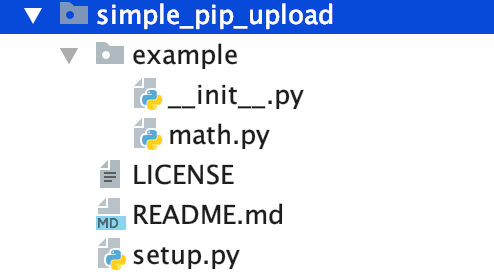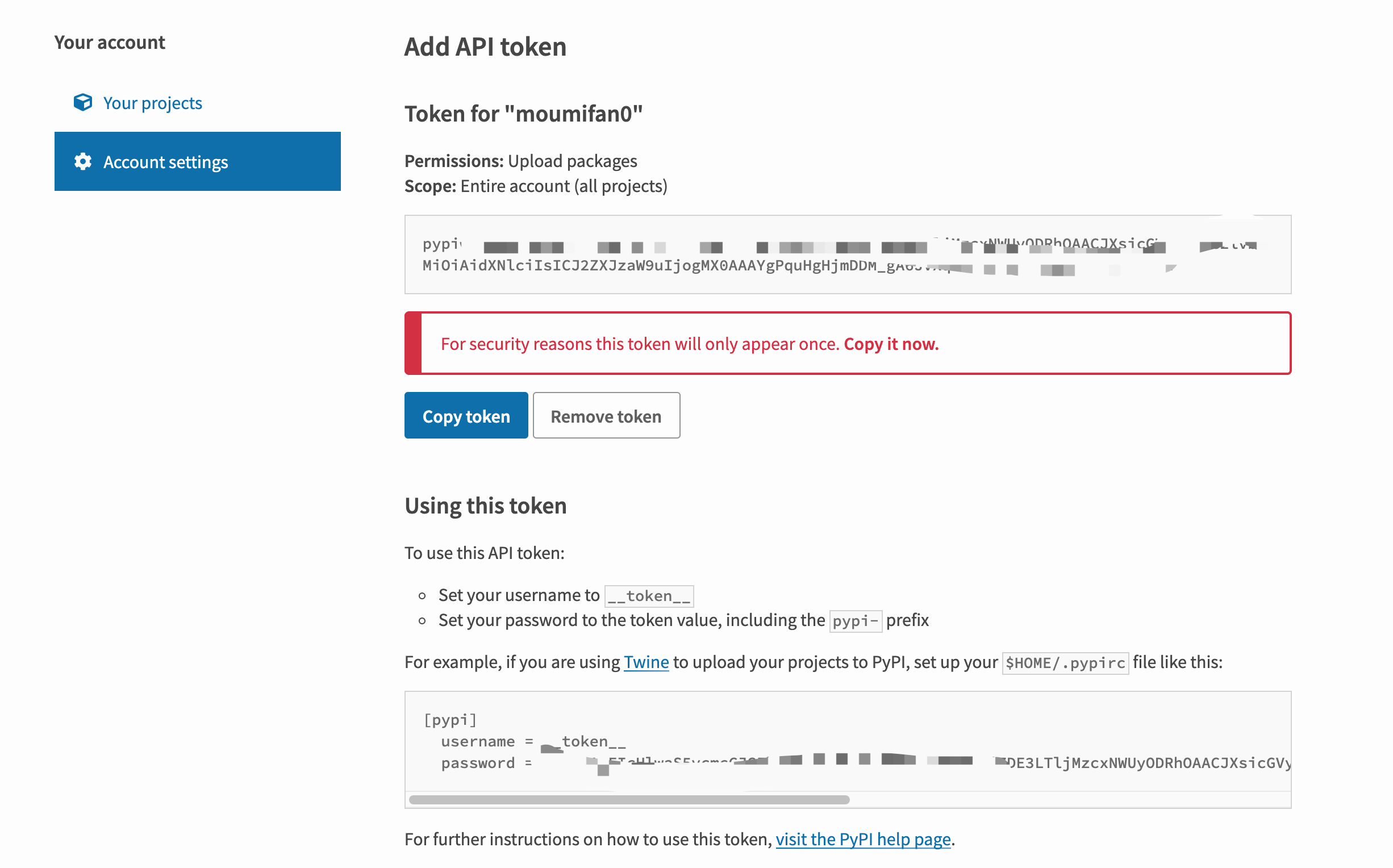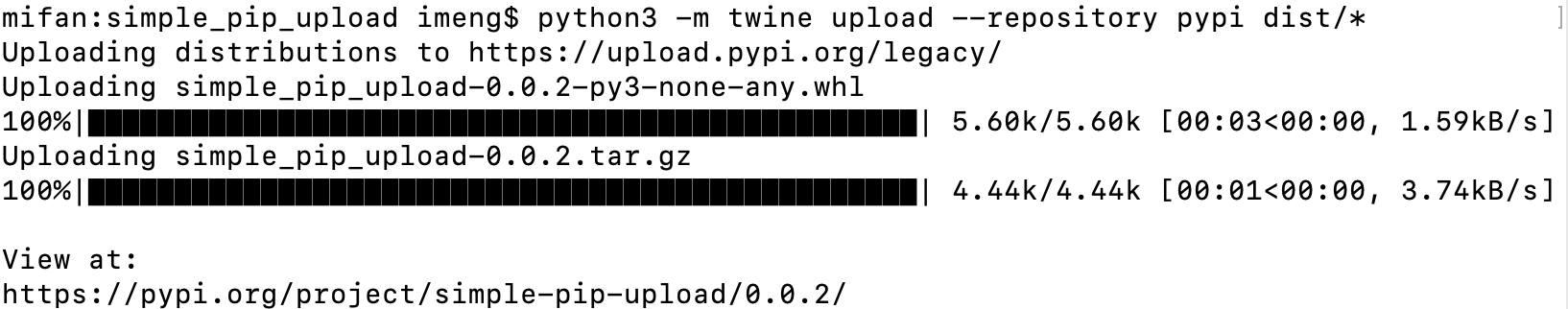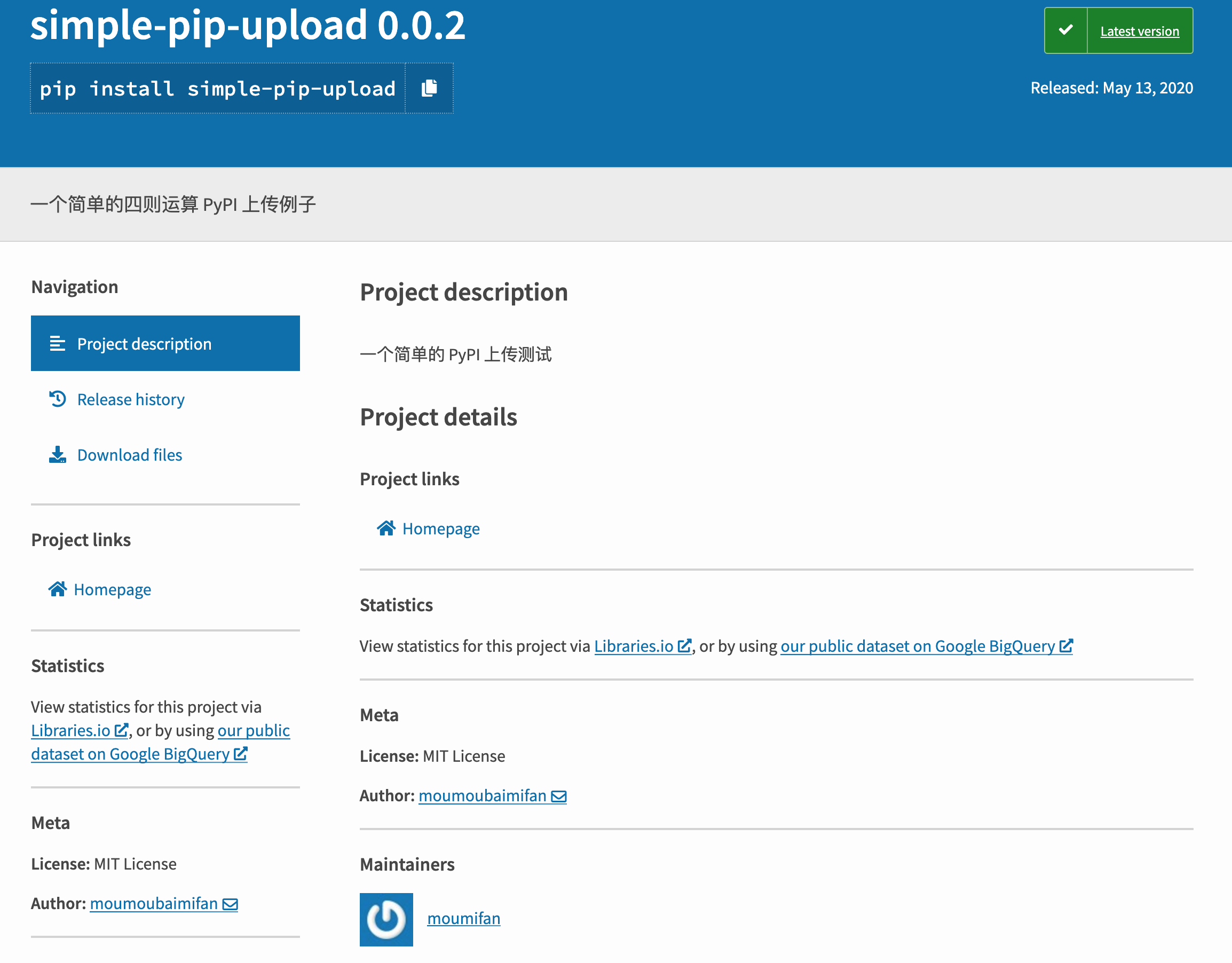# 发布代码到 PyPI## PyPI 概念

PyPI 全称 Python Package Index ，是一个 Python 的模块管理工具。提供对 Python 模块的查找、下载、安装、卸载等操作。

## 项目结构• example：模块名，代码写法: from example import math
• init.py：这里可以写代码，也可以是空文件
• math.py：代码模块，可以有多个 py 文件
• setup.py：setuptools 的构建脚本

# 编写代码

## 编写 math.py

math.py 里面只写了简单的加减乘除代码

``````def add(i, y):
print(str(i) + " + " + str(y) + " = " + str((i + y)))

def sub(i, y):
print(str(i) + " - " + str(y) + " = " + str((i - y)))

def mul(i, y):
print(str(i) + " * " + str(y) + " = " + str((i * y)))

def div(i, y):
print(str(i) + " / " + str(y) + " = " + str((i / y)))
``````

## 编写 setup.py

setup.py 是一个 setuptools 的构建脚本，其中包含了项目和代码文件的信息

``````import setuptools

setuptools.setup(
version="0.0.2",
author="moumoubaimifan",
author_email="example@example.com",
description="一个简单的四则运算 PyPI 上传例子",
long_description="一个简单的 PyPI 上传测试",
long_description_content_type="text/markdown",
url="https://github.com/JustDoPython/justdopython.github.io",
packages=setuptools.find_packages(),
classifiers=[
"Programming Language :: Python :: 3",
"Operating System :: OS Independent",
],
)
``````

setuptools.setup 的参数信息如下：

• name：项目的名称
• version：项目的版本
• author，author_email：作者和作者邮件
• description：项目的简单介绍
• long_description：项目的详细介绍
• long_description_content_type：表示用哪种方式显示long_description，一般是 md 方式
• url：项目的主页地址
• packages：项目中包含的子包，find_packages() 是自动发现根目录中的所有的子包。

## 编译

``````pip3 install --user --upgrade setuptools wheel
``````

``````python3 setup.py sdist bdist_wheel
``````

``````dist\
``````

## 上传PyPI 上传使用的是 Twine 模块，安装它

``````python3 -m pip install --user --upgrade twine
``````

``````python3 -m twine upload --repository pypi dist/*
``````## 使用模块

``````pip3 install simple-pip-upload
``````

``````>>> from example import math